# ؟ 5- How could you design a Three-Pass-Radix sort algorithm to sort the following group of...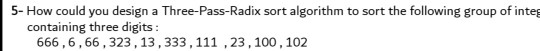؟

5- How could you design a Three-Pass-Radix sort algorithm to sort the following group of integ containing three digits: 666,6,66,323, 13, 333, 111, 23, 100, 102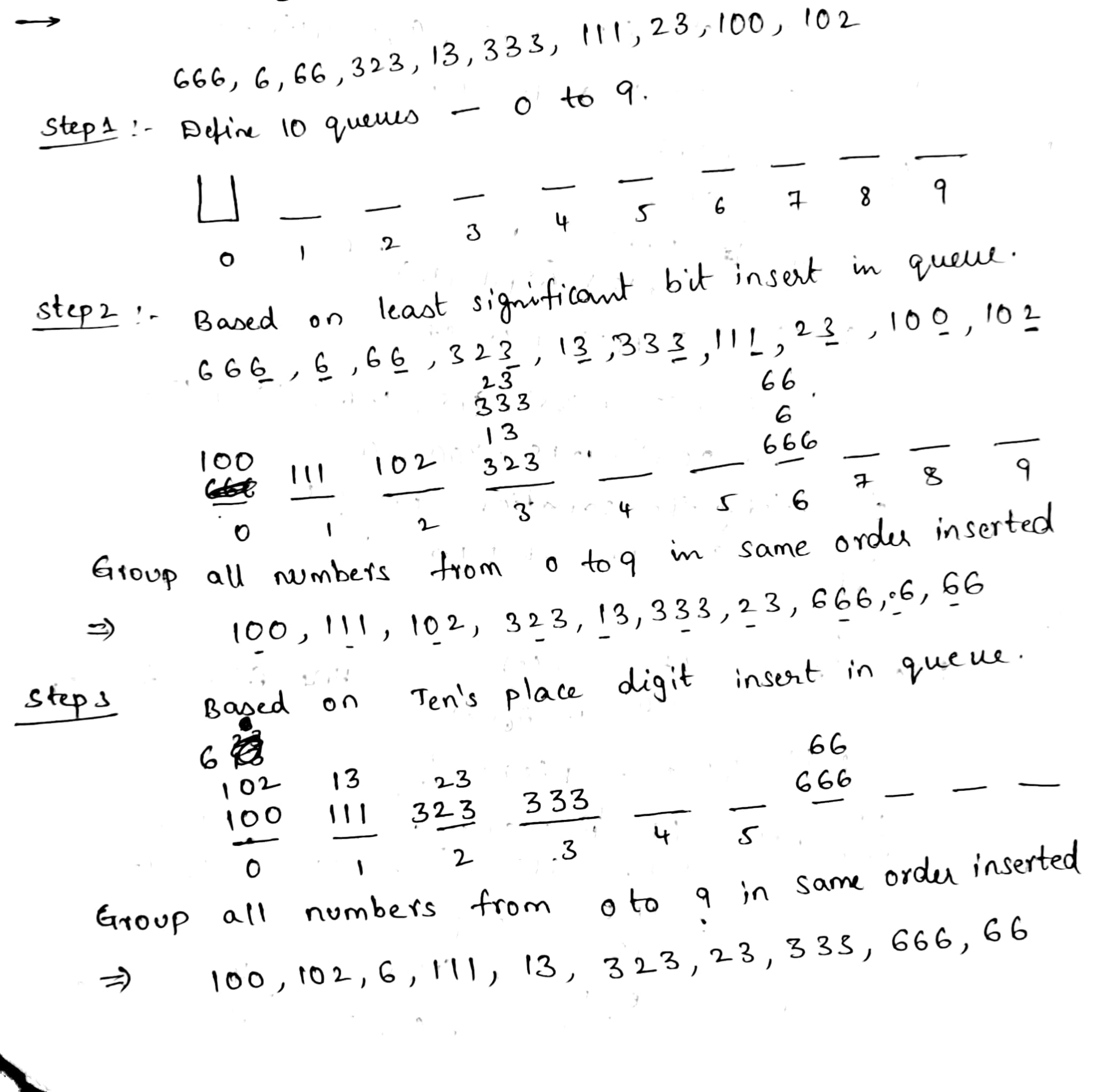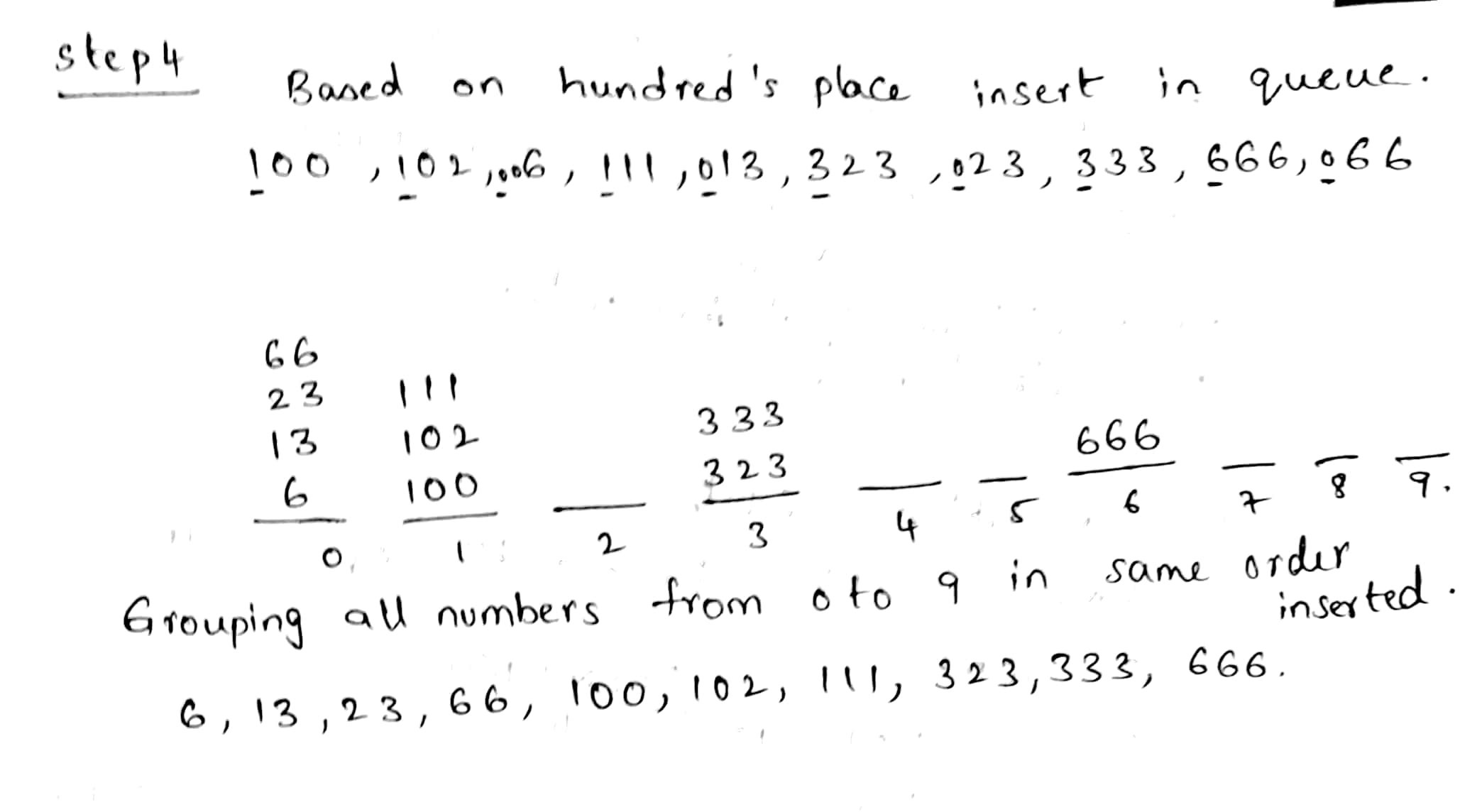///defining a function to which i pass the array list and size of n

void radixsort(int list[], int n) {
//get a max number for which the loop has to run i.e for 2 digit number we need to run 2 times i.e upto tens place

int m = getMax(list, n);

int exp;

//for every digit place i am calling function count sort
for (exp = 1; m / exp > 0; exp *= 10)
countSort(list, n, exp);
}

//getting the maxvalue from array

int getMax(int list[], int n) {
int mx = list;
int i;
for (i = 1; i < n; i++)
if (list[i] > mx)
mx = list[i];
return mx;
}

//function to insert the elements in queue based on digit --i.e units digit and tens place

void countSort(int list[], int n, int exp) {
//defining the output queue and of size n

int output[n];
int i, count = { 0 };

//loop to count the number of elements in the particular queue place ..i.e at queue 1 how many elements are //inserted

for (i = 0; i < n; i++)
count[(list[i] / exp) % 10]++;

for (i = 1; i < 10; i++)
count[i] += count[i - 1];

///inserting in the queue based on digits place in the queue output

for (i = n - 1; i >= 0; i--) {
output[count[(list[i] / exp) % 10] - 1] = list[i];
count[(list[i] / exp) % 10]--;
}

//coping the elements  and modifing the queue--list

for (i = 0; i < n; i++)
list[i] = output[i];
}

c code:

#include <stdio.h>
#include <conio.h>
#include <stdlib.h>

int getMax(int list[], int n) {
int mx = list;
int i;
for (i = 1; i < n; i++)
if (list[i] > mx)
mx = list[i];
return mx;
}

void countSort(int list[], int n, int exp) {
int output[n];
int i, count = { 0 };

for (i = 0; i < n; i++)
count[(list[i] / exp) % 10]++;

for (i = 1; i < 10; i++)
count[i] += count[i - 1];

for (i = n - 1; i >= 0; i--) {
output[count[(list[i] / exp) % 10] - 1] = list[i];
count[(list[i] / exp) % 10]--;
}

for (i = 0; i < n; i++)
list[i] = output[i];
}

void radixsort(int list[], int n) {
int m = getMax(list, n);

int exp;
for (exp = 1; m / exp > 0; exp *= 10)
countSort(list, n, exp);
}

void print(int list[], int n) {
int i;
for (i = 0; i < n; i++)
printf("%d\t", list[i]);
}

int main()
{
//defining the array list
int list[] = {666,6, 66, 323, 13, 333, 111, 23, 100,102 };
int i, n = sizeof(list) / sizeof(list);

printf("List of numbers before sort: \n");
for(i = 0; i<10; i++)
printf("%d\t", list[i] );
//calling the function

printf("\n\nList of numbers after sort: \n");
print(list, n);
printf("\n\n");
return 0;
}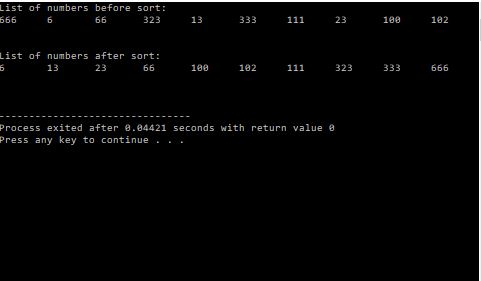##### Add Answer to: ؟ 5- How could you design a Three-Pass-Radix sort algorithm to sort the following group of...
Similar Homework Help Questions
• ### 5. Radix Sort Sort the following array using a radix sort with base 10, showing each...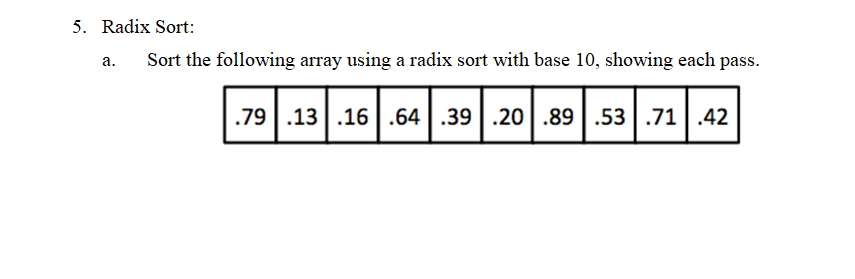5. Radix Sort Sort the following array using a radix sort with base 10, showing each pass a. .791316.64|.39|.20|.89.53|.71.42 5. Radix Sort Sort the following array using a radix sort with base 10, showing each pass a. .791316.64|.39|.20|.89.53|.71.42

• ### 5. Radix Sort Sort the following array using a radix sort with base 10, showing each...5. Radix Sort Sort the following array using a radix sort with base 10, showing each pass a. .791316.64|.39|.20|.89.53|.71.42

• ### 7. (14 points) Use LSD-first Radix Sort algorithm to sort the following array of numbers. Write...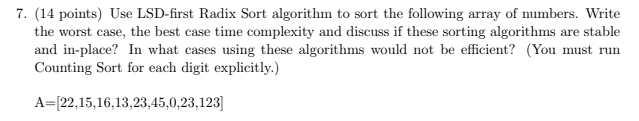7. (14 points) Use LSD-first Radix Sort algorithm to sort the following array of numbers. Write the worst case, the best case time complexity and discuss if these sorting algorithms are stable and in-place? In what cases using these algorithms would not be efficient? (You must run Counting Sort for each digit explicitly.) A=[22,15,16,13,23,45,0,23,123]

• ### Problem: Discuss in detail a data structure that on the radix sort algorithm. You will do this in the form of a 4-6 page paper. Your paper should discuss the underlying principle driving the data stru...

Problem: Discuss in detail a data structure that on the radix sort algorithm. You will do this in the form of a 4-6 page paper. Your paper should discuss the underlying principle driving the data structure, how it works (i.e. how elementary operations are performed/implemented on it), special features if any, and most importantly it's algorithmic analysis (Big-Oh cost) of all the key operations. For the time complexity you must discuss why the cost is O(?) instead of simply reporting...

• ### 7. Sort the following list into lexicographic order using a three-pass bucket sort: 521, 432, 743...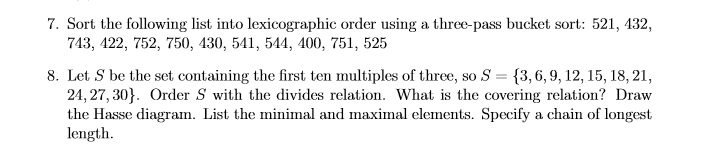7. Sort the following list into lexicographic order using a three-pass bucket sort: 521, 432, 743, 422, 752, 750, 430, 541, 544, 400, 751, 525 8. Let S be the set containing the first ten multiples of three, so S 3,6,9,12, 15, 18, 21, 24,27,30]. Order S with the divides relation. What is the covering relation? Draw the Hasse diagram. List the minimal and maximal elements. Specify a chain of longest length 7. Sort the following list into lexicographic order...

• ### 8. Design a flowchart for sorting the following numbers using "Bubble Sort" algorithm. (20) 74 101 5 [74 10 1 5...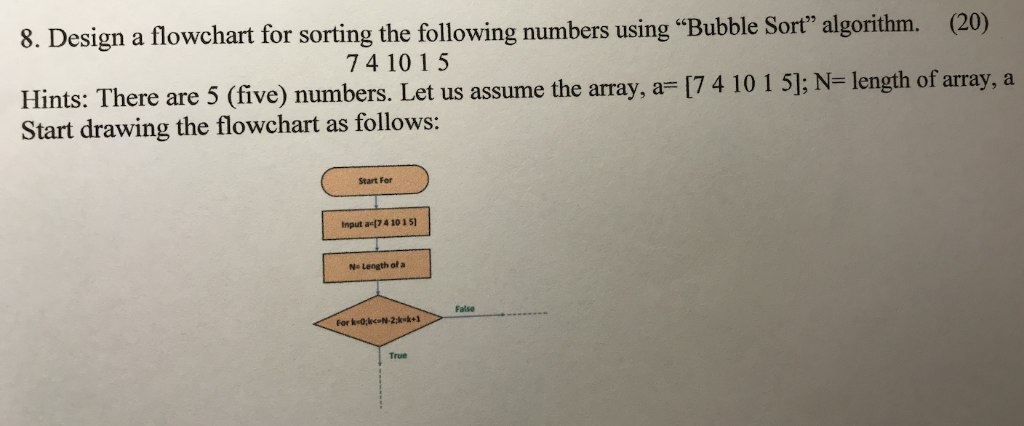8. Design a flowchart for sorting the following numbers using "Bubble Sort" algorithm. (20) 74 101 5 [74 10 1 5; N= length of array, a Hints: There are 5 (five) numbers. Let us assume the array, a Start drawing the flowchart as follows: Start For Input ac[74 10 1 5) Length of a False For k-0kcN-2:k-k+1 True 8. Design a flowchart for sorting the following numbers using "Bubble Sort" algorithm. (20) 74 101 5 [74 10 1 5; N=...

• ### You are given a list of strings L = {s1,s2 ...sn}. Each si is a string...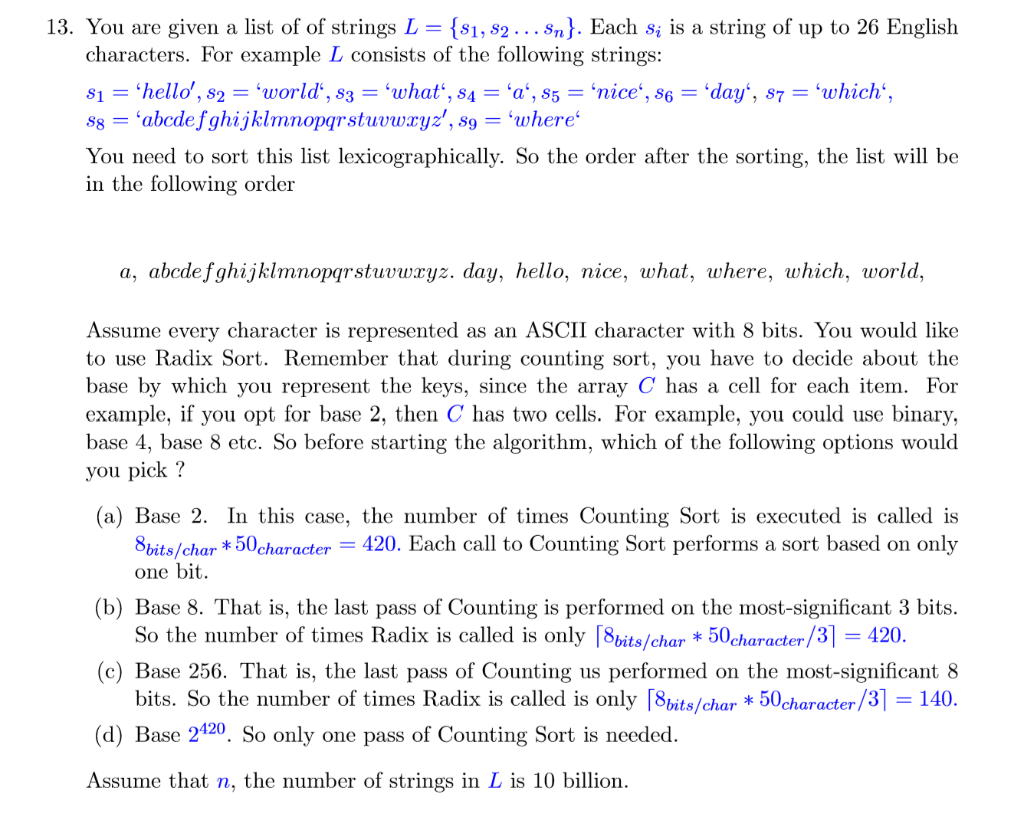You are given a list of strings L = {s1,s2 ...sn}. Each si is a string of up to 26 English characters. For example, L consists of the following strings: {s1, 82... Sn}. Each s; is a string of up to 26 English 13. You are given a list of of strings L = characters. For example L consists of the following strings: S1 =hello', S2 = 'world, s3 = 'what', s4 = 'a', 85 = 'nice', s6 = 'day',...

• ### Sorting Sort the following array using the quick sort algorithm: (4 Marks) a. 12 26 8...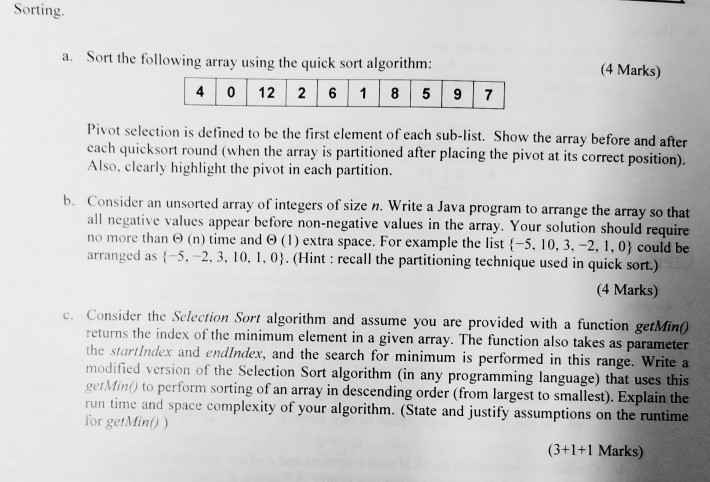Sorting Sort the following array using the quick sort algorithm: (4 Marks) a. 12 26 8 9 7 0 4 Pivot selection is defined to be the first element of each sub-list. Show the array before and after each quicksort round (when the array is partitioned after placing the pivot at its correct position). Also, clearly highlight the pivot in each partition b. Consider an unsorted array of integers of size n. Write a Java program to arrange the array...

• ### Design a program that allows you to experiment with different sort algorithms in Java. This program should allow you to...

Design a program that allows you to experiment with different sort algorithms in Java. This program should allow you to easily plug-in new sort algorithms and compare them. Assume that input data is generated randomly and stored in a text file (have no less than 2000 items to sort). Do not restrict your program to only one data type, or to one ordering relationship. The data type, ordering relationship, and the sorting method must be input parameters for your program....

• ### Question 3 Given the input list [72, 29, 18, 4, 5, 64, 37, 2, 13, 10,...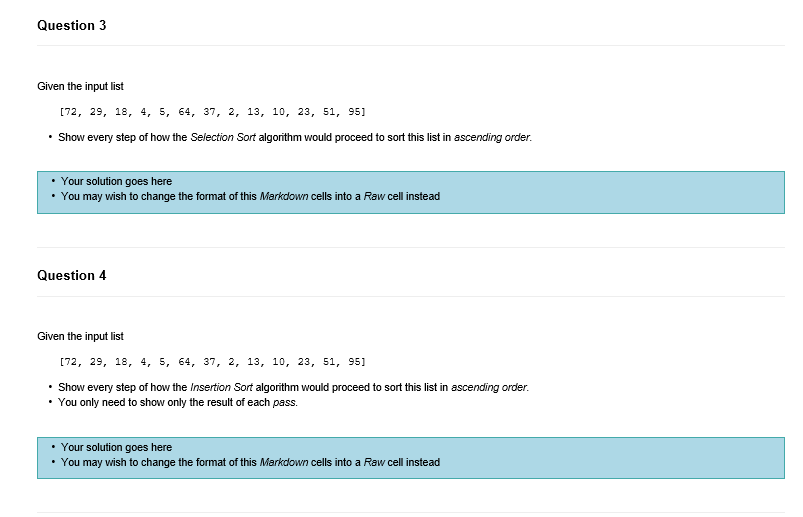Question 3 Given the input list [72, 29, 18, 4, 5, 64, 37, 2, 13, 10, 23, 51, 95] Show every step of how the Selection Sort algorithm would proceed to sort this list in ascending order. Your solution goes here • You may wish to change the format of this Markdown cells into a Raw cell instead Question 4 Given the input list [72, 29, 18, 4, 5, 64, 37, 2, 13, 10, 23, 51, 95] • Show every...

Free Homework App